# Overview

IKONOS is a commercial earth observation satellite. Details about the sensor are provided at Digital Globe's IKONOS Data Sheet. Wikipedia's article on IKONOS provides a nice overview as well.

## Modules overview

### Outside of GRASS

Satellite imagery can be managed and, at some extent, pre-processed with various GDAL utilities.

### Inside GRASS GIS

GRASS GIS features a complete set of modules and various add-ons enabling pre- and post-processing of IKONOS satellite imagery. The following lists offer an overview of related modules and add-ons.

## Pre-Processing

### Overview

Typically, multispectral satellite data are converted into physical quantities such as Radiance or Reflectance before they are subjected in multispectral analysis techniques (image interpretation, band arithmetic, vegetation indices, matrix transformations, etc.). The latter can be differentiated in Top of Atmosphere Reflectance (ToAR) which does not account for atmospheric effects (absorption or scattering) and in Top of Canopy Reflectance (ToCR) which introduces a "correction" for atmospheric effects.

In order to derive Reflectance values, likewise as with remotely sensed data acquired by other sensors, IKONOS raw image digital numbers (DNs) need to be converted to at-sensor spectral Radiance values. At-sensor spectral Radiance values are an important input for the equation to derive Reflectance values. Note, Spectal Radiance is the measure of the quantity of radiation that hits the sensor and typically expressed in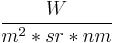$\frac{W}{m^2*sr*nm}$, that is watts per unit source area, per unit solid angle, and per unit wavelength.

• Converting DNs to at-sensor Radiance can be done by using the following equation:$L\lambda = \frac{10^4 * DN\lambda}{CalCoef\lambda * Bandwidth\lambda}$
• Converting to Top of Atmosphere Reflectance, also referred to as Planetary Reflectance, can be done by using the following equation:$\rho_p = \frac{\pi * L\lambda * d^2}{ESUN\lambda * cos(\Theta_S)}$
where:
•$\rho$ - Unitless Planetary Reflectance
•$\pi$ - mathematical constant (3.14159265358)
•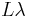$L\lambda$ spectral Radiance at the sensor's aperture, from equation... ToADD
•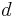$d$ - Earth-Sun distance in astronomical units, interpolated values
•$Esun$ - Mean solar exoatmospheric irradiance(s) (W/m2/μm), interpolated values
•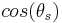$cos(\theta_s)$ - Solar_zenith_angle from the image acquisition's metadata

### Importing data

#### Importing

In the following example the publicly available IKONOS acqisition po_58204_0000000.20001116.China-Sichuan is used.

• Location creation based on georeferenced data

Once inside a Location that is defined by the spatial reference system in which the bands of interest are projected, they can be imported with the r.in.gdal module.

For example, GeoTIFF files can be imported by looping r.in.gdal over all of them

for TIF in echo *.tif; do r.in.gdal in=${TIF} out=${TIF%%.*}; done


To keep the raw material untouched, we create another Mapset inside the same Location and copy over the bands giving, optionally at the same time, a new name for each.

g.mapset -c pre-processing
g.copy rast=po_58204_blu_0000000,Blue_DNs
g.copy rast=po_58204_grn_0000000,Green_DNs
g.copy rast=po_58204_red_0000000,Red_DNs
g.copy rast=po_58204_nir_0000000,NIR_DNs
g.copy rast=po_58204_pan_0000000,Pan_DNs


### Deriving Physical Quantities

Conversions are implemented in a GRASS module available at https://github.com/NikosAlexandris/i.ikonos.toar. Detailed descriptions below.

Converting Digital Numbers to Radiance/Reflectance requires knowledge about the sensor's specific spectral band parameters. Those are, as extracted from the document IKONOS Planetary Reflectance and Mean Solar Exoatmospheric Irradiance, by Martin Taylor (see references):

 Pan_CalCoef=161	;	Pan_Width=403		;	Pan_Esun=1375.8
Blue_CalCoef=728	;	Blue_Width=71.3		;	Blue_Esun=1930.9
Green_CalCoef=720	;	Green_Width=88.6	;	Green_Esun=1854.8
Red_CalCoef=949	;	Red_Width=65.8		;	Red_Esun=1556.5
NIR_CalCoef=843	;	NIR_Width=95.4		;	NIR_Esun=1156.9


The following examples exemplify the conversion of raw Blue band digital numbers into Radiance and Reflectance.

Converting a Blue Band (Digital Numbers) in to Spectral at-sensor Radiance in the correct units to be further used for the conversion in to unitless Reflectance:

# set the region
g.region rast=Blue_DNs -p

# convert DNs to spectral Radiance values
r.mapcalc "Blue_Radiance = ( (10000 * IKONOS_Blue_DNs) / (728 * 71.3) )"


#### Planetary Reflectance

The equation to derive Reflectance values incorporates in addition:

• The mathematical constant Pi PI=3.14159265358.
• The Earth-Sun distance in astronomical units which depends on the acquisition's day of year (DOY -- also referred to as Julian day, Ordinal_date) and can be retrieved from the following spreadsheet <http://landsathandbook.gsfc.nasa.gov/excel_docs/d.xls>.
• The mean solar exoatmospheric irradiance in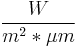$\frac{W}{m^2*\mu m}$. See 3rd column of (interplated) values given above.
• The cosine of the Solar Zenith Angle (SZA) at the time of the acquisition. The SZA can be calculated from its complementary Solar Elevation Angle (SEA) given in the image acquisition's metadata.

In the following example we accept as the acquisition's DOY=166 and SEA=52.78880. Hence, we get the Earth-Sun distance ESD=1.0157675 and SZA = 37.21120 deg.

Converting the in-Blue spectral band at-sensor Radiance in to Planerary Reflectance:

PI=3.14159265358; ESD=1.0157675; BAND_Esun=1930.9; SZA=37.21120
r.mapcalc "Blue_Reflectance = ( ${PI} * Blue_Radiance *${ESD}^2 ) / ( ${BAND_Esun} * cos(${SZA}) )"


#### Automatising Conversions

The conversion process can be scripted to avoid repeating the same steps for each band separately.

##### Python

A custom python script, performing the operations of interest, might be like i.ikonos.toar (for GRASS 7.x)

##### Bash

In bash, such a script might be as the following example. Note, however, in this example script constants, band parameters and acquisition related metadata are hard-coded!

#!/bin/bash

Pan_CalCoef=161		;	Pan_Width=403		;	Pan_Esun=1375.8
Blue_CalCoef=728	;	Blue_Width=71.3		;	Blue_Esun=1930.9
Green_CalCoef=720	;	Green_Width=88.6	;	Green_Esun=1854.8
Red_CalCoef=949		;	Red_Width=65.8		;	Red_Esun=1556.5
NIR_CalCoef=843		;	NIR_Width=95.4		;	NIR_Esun=1156.9

# set constants, band parameters and acquisition related metadata here!

# Pi, first 11 decimals
PI=3.14159265358

# Acquisition's Day of Year and estimated Earth-Sun Distance
DOY=166; ESD=1.0157675

# Sun Zenith Angle based on the acquisition's Sun Elevation Angle
SEA=52.78880; SZA=$(echo "90 -${SEA}" | bc )

# bands to process
Spectral_Bands="Pan Blue Green Red NIR"

# loop over all bands
for BAND in ${Spectral_Bands}; do echo "Processing the${BAND} spectral band"

# set region
g.region rast=${BAND}_DNs # set band parameters as variables eval BAND_CalCoef="${BAND}_CalCoef"
eval BAND_Width="${BAND}_Width"; echo "Band Parameters set to CalCoef=${!BAND_CalCoef}, Bandwidth=${!BAND_Width}" # conversion to Radiance r.mapcalc "${BAND}_Radiance = ( ( 10^4 * ${BAND}_DNs ) / (${!BAND_CalCoef} * ${!BAND_Width} ) )" # add info r.support map=${BAND}_Radiance \
title="" \
units="W / m2 / μm / ster" \
description="At-sensor echo ${BAND} band spectral Radiance (W/m2/μm/sr)" \ source1='"IKONOS Planetary Reflectance and Mean Solar Exoatmospheric Irradiance", by Martin Taylor, Geoeye' # set Earth-Sun distance eval BAND_Esun="${BAND}_Esun"; echo "Using Esun=${!BAND_Esun}" # calculate ToAR r.mapcalc "${BAND}_ToAR = \
( ${PI} *${BAND}_Radiance * ${ESD}^2 ) / (${!BAND_Esun} * cos(${SZA}) )" # add some metadata r.support map=${BAND}_ToAR \
title="echo ${BAND} band (Top of Atmosphere Reflectance)" \ units="Unitless" \ description="Top of Atmosphere echo${BAND} band spectral Reflectance (unitless)" \
source1='"IKONOS Planetary Reflectance and Mean Solar Exoatmospheric Irradiance", by Martin Taylor, Geoeye' \
source2="e.g., the Image Provider!" \
history="PI=3.14159265358; ESD=1.0157675; BAND_Esun=1930.9; SZA=37.21120"

done


## Post-Processing

Having beforehand satellite image data expressed in physical quantities (radiance or reflectance) is preferred to follow-up with digital image analysis techniques. A few common post-processing practices are Contrast-Enhancement, Pan-Sharpening and creating Pseudo- or True-Color Composites. Other well known enhancing manipualtions to support the analyses of satellite imagery, include deriving Vegetation Indices, transforming multi-spectral data based on PCA and Segmenting images.

### Pan-Sharpening

Pan-Sharpening / Fusion is the process of merging high-resolution panchromatic and lower resolution multi-spectral imagery. GRASS 7 holds a dedicated pan-sharpening module, i.pansharpen which features three techniques for sharpening, namely the Brovey transformation, the classical IHS method and one that is based on Principal Components Analysis (PCA). Another algorithm deriving excellent detail and a realistic representation of original multispectral scene colors, is the High-Pass Filter Addition (HPFA) technique. It is available through the add-on i.fusion.hpf (src) (for GRASS 6, please refer to a bash shell script https://github.com/NikosAlexandris/i.fusion.hpf.sh which is, however, unmaintained).

One approach inside GRASS-GIS to get an acceptable color-balanced composite image after Pan-sharpening 11-bit IKONOS spectral bands comprises the following steps

1. rescale the 11-bit IKONOS spectral bands to 8-bit ranging in [0, 255] (r.rescale)
2. pan-sharpen with any of the featured methods (Brovey, IHS, PCA) (i.pansharpen)
3. color-balance by using the i.landsat.rgb module or manually adjusting the color tables of the bands of interest
4. create a composite image by using the r.compose module

#### Example Instructions for i.pansharpen

i.pansharpen works fine with 8-bit raster maps as an input. If the data to be processed are out of this range, that is out of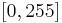$[0, 255]$, they can be rescaled to fit into this range by using GRASS' r.rescale module.

Given an IKONOS set of 11-bit spectral bands (Blue, Green, Red, NIR and Pan) ranging between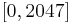$[0, 2047]$, and then querying for example the Blue band r.info Blue_DNs -r, would return

min=0
max=2047

##### Rescaling single bands

Rescaling the Blue band to range between [0, 255]

r.rescale in=Blue_DNs out=Blue_DNs_255 from=0,2047 to=0,255


The same step applies to both the rest of the multi-spectral bands and the Panchromatic band of interest. If working under Bash, To repeat the same command (given, it was the last on used in the GRASS' terminal), one can isntruct

!!:gs/Blue/Green


This would substitute everywhere found on the last command the string "Blue" with the string "Green" and re-execute it. The same can be done for the rest of the bands, namely Red, NIR and Pan. Some attention is required to instruct substitution of the last used string.

##### Rescaling all bands at once

Of course, one can always use a for loop

for DN in g.mlist rast pat=*DNs; do r.rescale in=${DN} out=${DN}_255 from=0,2047 to=0,255; done

##### Pan-Sharpening

As usual when working with GRASS, it is required to set the region of interest, i.e. g.region rast=Blue_DNs_255 to match the extent of the band(s) or else. The resolution itself is taken care in this particular case by the module and the resulting pan-sharpened raster maps will of the same high(er) resolution as the Panchromatic band.

An example command for an IHS-based Pan-Sharpening action might look like

i.pansharpen pan=Pan_DNs_255 ms1=Blue_DNs_255 ms2=Green_DNs_255 ms3=Red_DNs_255 output=sharptest255 sharpen=ihs

##### Color Re-Balancnig

After the process completion, the module outputs

...
The following pan-sharpened output maps have been generated:
sharptest255_red
sharptest255_green
sharptest255_blue

To visualize output, run: g.region -p rast=sharptest255.red
d.rgb r=sharptest255_red g=sharptest255_green b=sharptest255_blue

If desired, combine channels into a single RGB map with 'r.composite'.


Normally it should be enough to re-balance the colors after the pan-sharpening action by using for example the i.landsat.rgb module or manual adjustment of each of the three bands that would compose an RGB image.

i.landsat.rgb r=sharptest255_red g=sharptest255_green b=sharptest255_blue -p

##### Automatising Sharpening & RGB Composition

An experimental bash script to automatise the sharpening/fusion and RGB composition process is demonstrated here. The script uses the Spectral Reflectance values (double precision values), gained from previous steps (see above), and produces Pan-sharpened images by applying all of the three methods that Template:Addon offers. In addition, it attempts to produce True-Color composite images, without and with re-balancing the color tables by using the i.landsat.rgb module.

Initial tests indicate that at least one of the three sharpening methods, combined with re-balancing, produces very clear and balanced True-Color composites. Note, there is no 100% confidence that it will produce nice looking True-Color composites. Some of the methods might simply work, others might deliver fancy-colored images. As usual, some manual action might be required to get the re-balancing to work as best as possible.

The script can be expanded in terms of using more inputs by utilising bash's positional parameters. However, care has to be taken to alter the instruction that concerns the conversion from double precision values to 8-bit integers.

#!/bash/sh

# use in G7 only :-)

echo "Rescaling all images to 8-bit"
# convert to 8-bit first :-!
for Method_Input in \
"Pan ToAR" \
"Blue ToAR" \
"Green ToAR" \
"Red ToAR" \
"NIR ToAR"

do

# parse "${Method_STR}" and set positional parameters set --$Method_Input ; echo -e "\n" #echo $1$2

# input BAND is...
eval BAND="${1}_${2}"

# set region
g.region rast=${BAND} # integerise r.mapcalc --v \ "${BAND}_integerised = round( 1000000 * ${BAND} )" # how many decimals? # integerised BAND is... eval BAND="${1}_${2}_integerised" # get${min} and ${max} eval r.info -r${BAND}

#
r.rescale in=${1}_${2}_integerised out=${1}_${2}_255 from=${min},${max} to=0,255

done ########################################################################
echo "Rescaling done!"

# pan-sharpen High Resolution imagery ---------------------------------------
# needs setting some naming conventions
for Method_Input in \
"ihs ToAR" \
"brovey ToAR" \
"pca ToAR"

do

# parse "${Method_STR}" and set positional parameters set --$Method_Input ; echo $1$2

# some echo
echo "Pan-Sharpening the <${2}> images based on the <${1}> method"

i.pansharpen \
sharpen=${1} \ pan=Pan_${2}_255 \
ms1=Blue_${2}_255 \ ms2=Green_${2}_255 \
ms3=Red_${2}_255 \ output=sharp_${1}_${2}_255 done ######################################################################## # compose BGRs -------------------------------------------------------------- # loop over Methods and Input Types, compose RGBs for Method_Input in \ "ihs ToAR" \ "brovey ToAR" \ "pca ToAR" do # parse "${Method_STR}" and set positional parameters
set -- $Method_Input ; echo$1 $2 # region g.region rast=sharp_"${1}"_"${2}"_255_blue # compose r.composite --o \ r=sharp_"${1}"_"${2}"_255_red \ g=sharp_"${1}"_"${2}"_255_green \ b=sharp_"${1}"_"${2}"_255_blue \ out=rgb_sharpened_"${1}"_"${2}"_255 done ####################################################################### # re-balance & re-compose colors -------------------------------------------- for Method_Input in \ "ihs ToAR" \ "brovey ToAR" \ "pca ToAR" do # parse "${Method_STR}" and set positional parameters
set -- $Method_Input ; echo$1 $2 # re-balance i.landsat.rgb -p \ r=sharp_"${1}"_"${2}"_255_red \ g=sharp_"${1}"_"${2}"_255_green \ b=sharp_"${1}"_"${2}"_255_blue # region -- Really necessary, again? g.region rast=sharp_"${1}"_"${2}"_255_blue # re-compose r.composite --o \ r=sharp_"${1}"_"${2}"_255_red \ g=sharp_"${1}"_"${2}"_255_green \ b=sharp_"${1}"_"${2}"_255_blue \ out=rgb_sharpened_"${1}"_"\${2}"_255_rebalanced

done ########################################################################


#### Pan-Sharpening based on the HPFA technique

The technique, implemented via the GRASS add-on i.fusion.hpf (src), as indicated above, involves a convolution using a High Pass Filter (HPF) on the high resolution data, then combining this with the lower resolution multispectral data.

Source: "Optimizing the High-Pass Filter Addition Technique for Image Fusion", Ute G. Gangkofner, Pushkar S. Pradhan, and Derrold W. Holcomb (2008)

The algorithm's steps are:

1. Computing the ratio of the low (Multi-Spectral) to the high (Panchromatic) resolution
2. High Pass Filtering the Panchromatic Image
3. Resampling the Multi-Spectral image to the higher resolution
4. Adding a weighted High-Pass-Filtered image to the upsampled Multi-Spectral image
5. Optionally, matching the histogram of the Pan-Sharpened image to the one of the original Multi-Spectral image